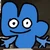## FANDOM

2,830 Pages# Alemagno12

## aka Ale/Nish

0 Discussion posts
My favorite wikis
• I live in yes
• My occupation is yes
• I am yes
•## Hierarchy of Stuff (Assigning levels to stuff according to what they contain)

May 14, 2017 by Alemagno12

C

l

o

s

e

d

•## Expressing dimensions using Polynomials

May 21, 2016 by Alemagno12

We notate the 0th dimension.

We have a line that is composed of 0-dimensional dots, so we will note the number of dots with n=n dots.

We notate the 1st dimension.

The limit of the first line will be notated as X. And dots in the next line will be denoted as X+n.

We have a plane that is composed of 1-dimensional lines, so we will note the number of lines with mX=m lines.

So for m lines and n dots on the m+1th line, we have mX+n.

We notate the 2nd dimension.

The limit of the first plane will be notated as X2. And dots+lines in the next plane will be denoted as X2+mX+n.

We have a cube that is composed of 2-dimensional planes, so we will note the number of planes with lX2=l planes.

So for l planes and m lines in the l+1th plane and n dots on the m+1th …

•## AAAAAAAAAAAAAAAAAAAAAAAAAA

March 25, 2016 by Alemagno12

So, uhh....

I can't focus on what I'm doing. There are of my hiatuses and of my unfinished ideas, and my attention is focused on Googology Wiki, so I can't do much.

I'm focusing on making a new dimensional index using X-structures (inspired by Holomanga). It will be rather simple so I can finish it quickly.

Maybe I will make some -verse ideas.

And so...........see you later!

-AM12

•## Dimensional notations

December 14, 2015 by Alemagno12

An attempt to explain easier my Dimensional Array Notation, with other dimensional notations by me.

The first notation we are gonna use is called Postix Notation.

First off, we need to define the dimensions for Postix Notation.

Most of us here know the concepts of dimensions, so i will go to explaining the first level of Postix Notation.

Each dimension is measured with an unit of length (denoted L).

The dimension number is determined with the unit D, so nD represents the nth dimension.

An expression of First-level Postix Notation is divided into measures, denoted as (nDmL). This one represents that the length of the nth dimension is mL.

An expression of First-level Postix Notation is denoted as (1DaL)(2DbL)(3DcL)...(nDzL), each measure determing t…

•## The NEW dimensional array notation

December 9, 2015 by Alemagno12

I have been redesigning my dimensional array notation, and i came up with a function D(a,b,c,...,x,y,z) to denote it. It can be extended to dimensional arrays, and even, dimensional array notation arrays.

This notation will be explained here.

The linear arrays are denoted D(a,b,c,...,x,y,z), and each of one represent the coordinates in the dimensions.

Case 1: In case of a linear array, The nth entry will represent the length in the nth dimension.

The next level of Dimensional Array Notation will be extending the array to multiple rows. The linear array only has 1 row. Rows are separated by |.

Normal dimensions are in the first hyperdimension. The first dimension of the nth hyperdimension will represent the first dimension above all dimensions o…